# 机器学习|层次聚类方法 (Python 语言描述)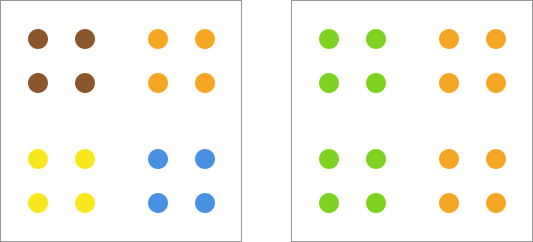• 自底向上层次聚类法：该方法的过程被称为「凝聚」(Agglomerative)，也就是把数据集中的每个元素看作是一个类别，然后进行迭代合并成为更大的类别，直到满足某个终止条件。

• 自顶向下层次聚类法：该方法的过程被称为「分裂」(Divisive)，也就是凝聚的反向过程。首先，把数据集看作是一个类别，然后递归地划分为多个较小的子类，直到满足某个终止条件。

## 自底向上层次聚类法

### 自底向上层次聚类流程

1. 将数据集中每个样本标记为 1 类，即 $D$ 初始时包含的类别（Class）为 C，$$C=\left ( {c_1,c_2,\cdots,c_n} \right )$$。
2. 计算并找出 C 中距离最近的 2 个类别，合并为 1 类。
3. 依次合并直到最后仅剩下一个列表，即建立起一颗完整的层次树。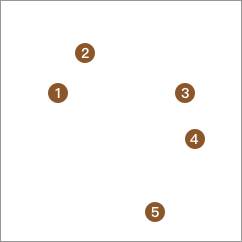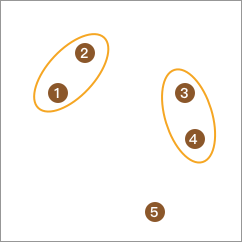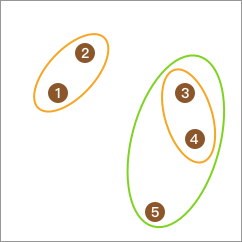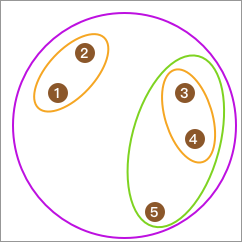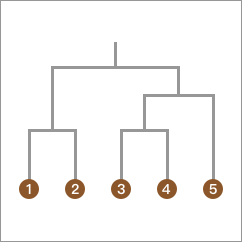### 距离计算方法

#### 单连接（Single-linkage）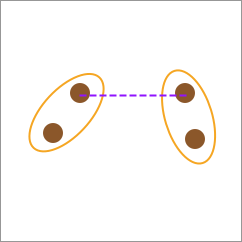#### 全连接（Complete-linkage）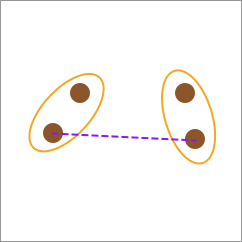#### 平均连接（Average-linkage）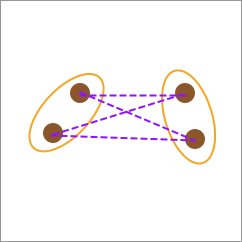#### 中心连接（Center-linkage）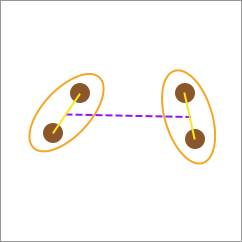### Agglomerative 聚类 Python 实现

(array([[  6.04774884, -10.30504657],
[  2.90159483,   5.42121526],
[  4.1575017 ,   3.89627276],
[  1.53636249,   5.11121453],
[  3.88101257,  -9.59334486],
[  1.70789903,   6.00435173],
[  5.69192445,  -9.47641249],
[  5.4307043 ,  -9.75956122],
[  5.85943906,  -8.38192364],
[  0.69523642,   3.23270535]]), array([0, 1, 1, 1, 0, 1, 0, 0, 0, 1]))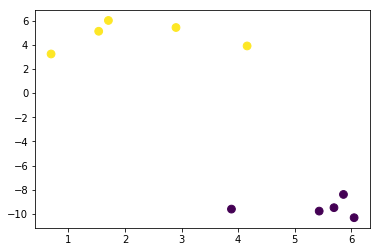☞ 第 1 次迭代

---

---

---

---

---

---

---

---

---

---

☞ 第 2 次迭代

---

---

---

---

---

---

---

---

---

☞ 第 3 次迭代

---

---

---

---

---

---

---

---

☞ 第 4 次迭代

---

---

---

---

---

---

---

☞ 第 5 次迭代

---

---

---

---

---

---

☞ 第 6 次迭代

---

---

---

---

---

☞ 第 7 次迭代

---

---

---

---

☞ 第 8 次迭代

---

---

---

☞ 第 9 次迭代

---

---

array([[ 3.40447914, -2.70986843]])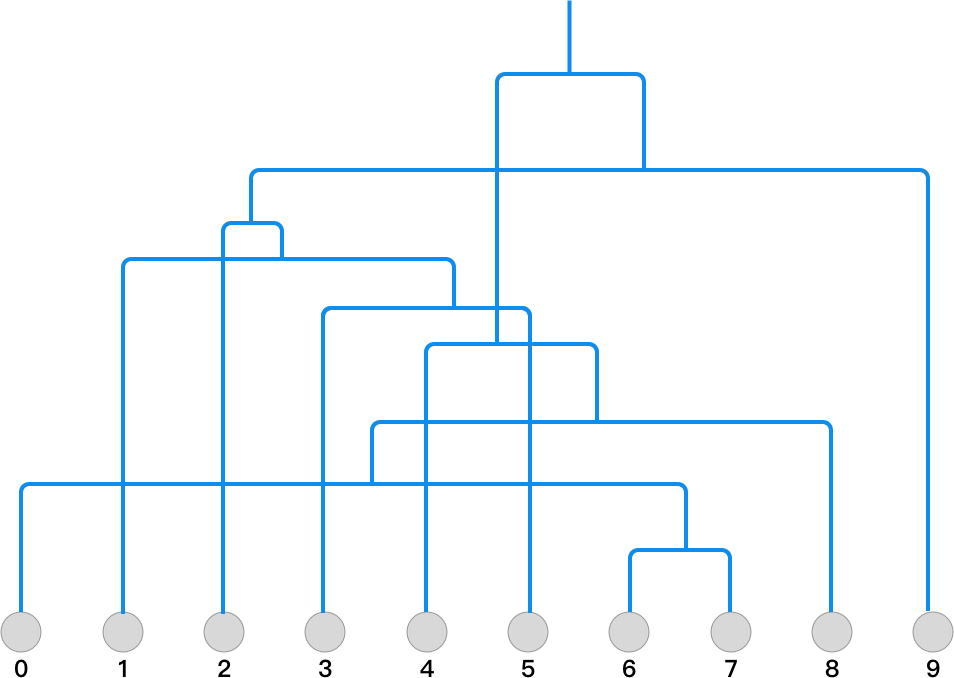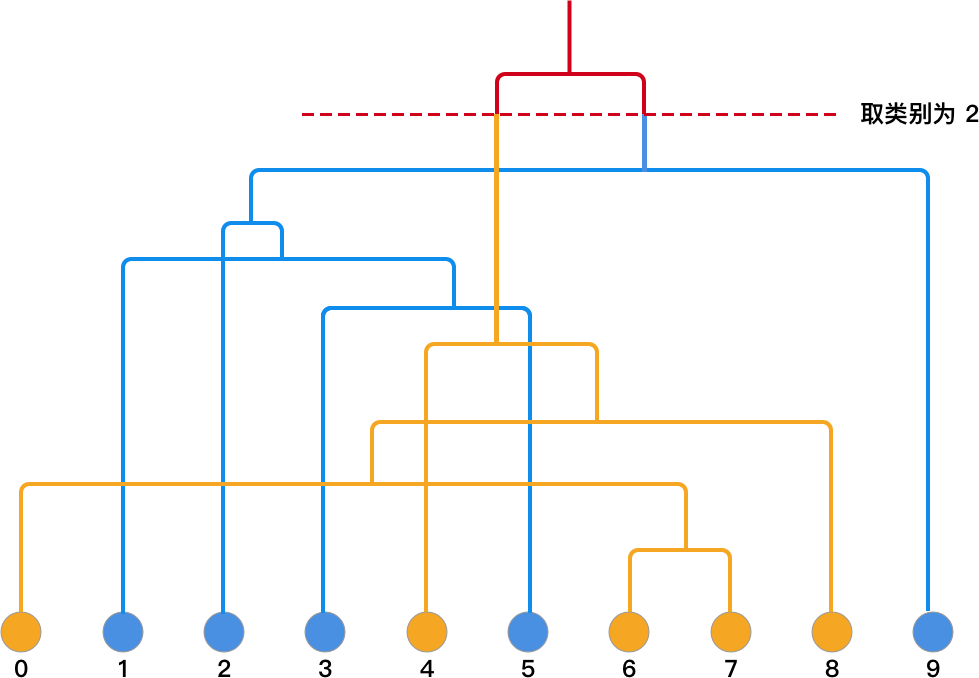### 使用 scikit-learn 完成 Agglomerative 聚类

scikit-learn 中也提供了 Agglomerative 聚类的类，相应的参数解释如下：

• n_clusters: 表示最终要查找类别的数量，例如上面的 2 类。
• affinity: 亲和力度量，有 euclidean（欧式距离）, l1（L1 范数）, l2（L2 范数）, manhattan（曼哈顿距离）等可选。
• linkage: 连接方法：ward（单连接）, complete（全连接）, average（平均连接）可选。

array([1, 0, 0, 0, 1, 0, 1, 1, 1, 0])


## 自顶向下层次聚类法

### 利用 K-Means 算法进行分割

1. 把数据集 D 归为单个类别 C 作为顶层。
2. 使用 K-Means 算法把 C 划分成 2 个子类别，构成子层；
3. 可递归使用 K-Means 算法继续划分子层到终止条件。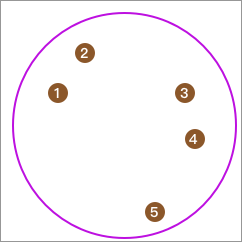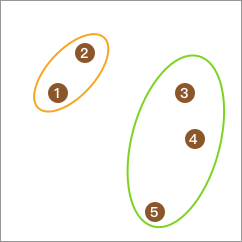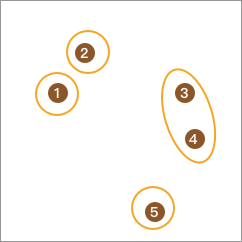### 利用平均距离进行分割

1. 把数据集 D 归为单个类别 C 作为顶层。
2. 从类别 C 中取出点 d，使得 d 满足到 C 中其他点的平均距离最远，构成类别 N。
3. 继续从类别 C 中取出点 d’, 使得 d’ 满足到 C 中其他点的平均距离与到 N 中点的平均距离之间的差值最大，并将点放入 N。
4. 重复步骤 3，直到差值为负数。
5. 再从子类中重复步骤 2,3,4 直到全部点单独成类，即完成分割。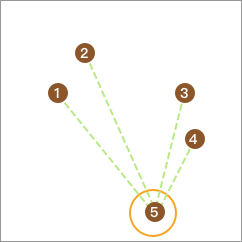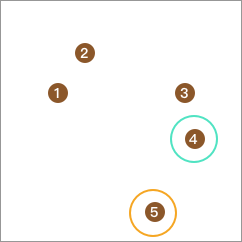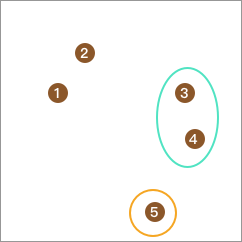## BIRCH 聚类算法

BIRCH 的全称为 Balanced Iterative Reducing and Clustering using Hierarchies，直译过来就是「使用层次方法的平衡迭代规约和聚类」。该算法由时任 IBM 工程师 Tian Zhang 于 1996 年发明，详见 论文

BIRCH 最大的特点就是高效，可用于大型数据集的快速聚类。

### CF 聚类特征

BIRCH 的聚类过程主要是涉及到 CF 聚类特征和 CF Tree 聚类特征树的概念。所以，我们需要先了解什么是聚类特征。

$$CF = \langle ( N, LS, SS ) \rangle$$

• N = 5
• $$LS = (1+2+1+7+8, 3+5+3+9+8) =(19, 28)$$
• $$SS = (1^2+2^2+1^2+7^2+8^2+3^2+5^2+3^2+9^2+8^2) = (307)$$

$$CF = \langle 5, (19,28), (307) \rangle$$

CF 拥有可加性，例如当 $$CF’= \langle 3, (35, 36), 857 \rangle$$ 时：

$$CF’ + CF = \langle 5, (19,28), (307) \rangle + \langle 3, (12, 26), 87 \rangle = \langle 8, (31, 54), (394) \rangle$$

CF 聚类特征本质上是定义类别（簇）的信息，并有效地对数据进行压缩。

### CF 聚类特征树

CF 树由根节点（root node）、枝节点（branch node）和叶节点（leaf node）构成。另包含有三个参数，分别为：枝平衡因子 $$\beta$$、叶平衡因子 $$\lambda$$ 和空间阈值 $$\tau$$。而非叶节点（nonleaf node）中包含不多于 $$\beta$$ 个 $$[CF,child_{i}]$$ 的元项。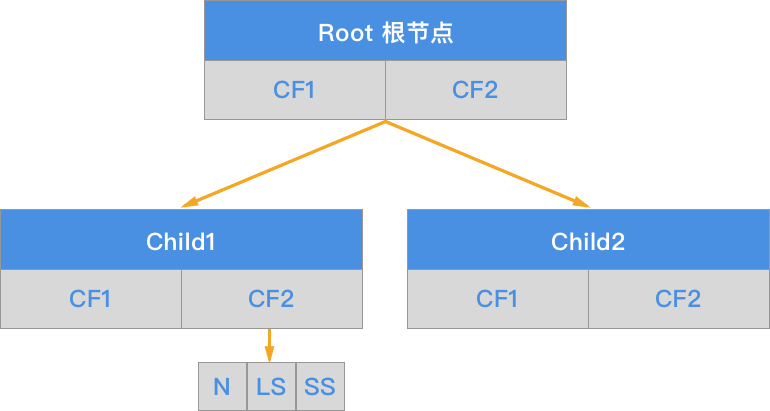BIRCH 算法的核心就是基于训练样本建立了 CF 聚类特征树。CF 聚类特征树对应的输出就是若干个 CF 节点，每个节点里的样本点就是一个聚类的类别。

1. BIRCH 算法在建立 CF 特征树时只存储原始数据的特征信息，并不需要存储原始数据信息，内存开销上更优，计算高效。
2. BIRCH 算法只需要遍历一遍原始数据，而 Agglomerative 算法在每次迭代都需要遍历一遍数据，再次突出 BIRCH 的高效性。
3. BIRCH 属于在线学习算法，并支持对流数据的聚类，开始聚类时并不需要知道所有的数据。

### BIRCH 聚类实现array([[ 0.,  0.,  5., 13.,  9.,  1.,  0.,  0.],
[ 0.,  0., 13., 15., 10., 15.,  5.,  0.],
[ 0.,  3., 15.,  2.,  0., 11.,  8.,  0.],
[ 0.,  4., 12.,  0.,  0.,  8.,  8.,  0.],
[ 0.,  5.,  8.,  0.,  0.,  9.,  8.,  0.],
[ 0.,  4., 11.,  0.,  1., 12.,  7.,  0.],
[ 0.,  2., 14.,  5., 10., 12.,  0.,  0.],
[ 0.,  0.,  6., 13., 10.,  0.,  0.,  0.]])


### PCA 主成分分析（降维）

1. 参考本文的目的，方便将数据用于低维空间可视化。聚类过程中的可视化是很有必要的。

2. 高维度数据集往往就意味着计算资源的大量消耗。通过对数据进行降维，我们就能在不较大影响结果的同时，减少模型学习时间。

1.对各维度特征进行标准化处理：

$$x_j^{(i)}=\frac{x_j^{(i)}-\mu_j}{s_j}$$

2.计算对应的协方差矩阵：
$$\Sigma = \frac{1}{m}\sum\limits_{i=1}^{m}(x^{(i)})(x^{(i)})^T = \frac{1}{m} \cdot X^TX$$

3.对协方差矩阵进行奇异值分解（SVD），得到特征向量：
$$(U,S,V^T) = SVD(\Sigma)$$

4.从 U 中取出前 m 个左奇异向量，构成一个约减矩阵 $$U_{reduce}$$ ：
$$U_{reduce} = (u^{(1)},u^{(2)},\cdots,u^{(k)})$$

5.计算新的特征向量 $$z^{(i)}$$：
$$z^{(i)}=U_{reduce}^T \cdot x^{(i)}$$

6.最后根据新的特征向量执行特征还原：
$$x_{new}=U_{reduce}z^{(i)}$$

PCA 的过程听起来简单，执行起来还是比较麻烦的。所以，我们这里直接使用 scikit-learn 中 PCA 方法完成：

• n_components= 表示需要保留主成分（特征）的数量。
• copy= 表示针对原始数据降维还是针对原始数据副本降维。当参数为 False 时，降维后的原始数据会发生改变，这里默认为 True。
• whiten= 白化表示将特征之间的相关性降低，并使得每个特征具有相同的方差。
• svd_solver= 表示奇异值分解 SVD 的方法。有 4 参数，分别是：auto, full, arpack, randomized

array([[ -1.25946556,  21.27488973],
[  7.95761389, -20.7686883 ],
[  6.99192015,  -9.95599952],
...,
[ 10.80128328,  -6.96025693],
[ -4.87209994,  12.42397329],
[ -0.34439245,   6.36553344]])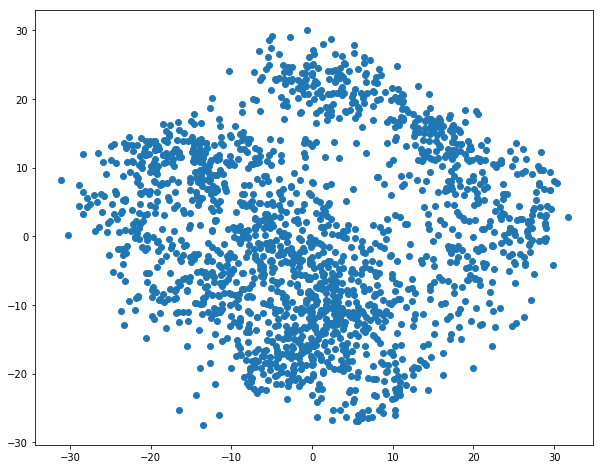BIRCH 在 scikit-learn 对应的主要类及参数如下：

• threshold: 每个 CF 的空间阈值 $$\tau$$。参数值越小，则 CF 特征树的规模会越大，学习时花费的时间和内存会越多。默认值是 0.5，但如果样本的方差较大，则一般需要增大这个默认值。
• branching_factor: CF 树中所有节点的最大 CF 数。该参数默认为 50，如果样本量非常大，一般需要增大这个默认值。
• n_clusters: 虽然层次聚类无需预先设定类别数量，但可以设定期望查询的类别数。

array([3, 0, 0, ..., 0, 5, 9])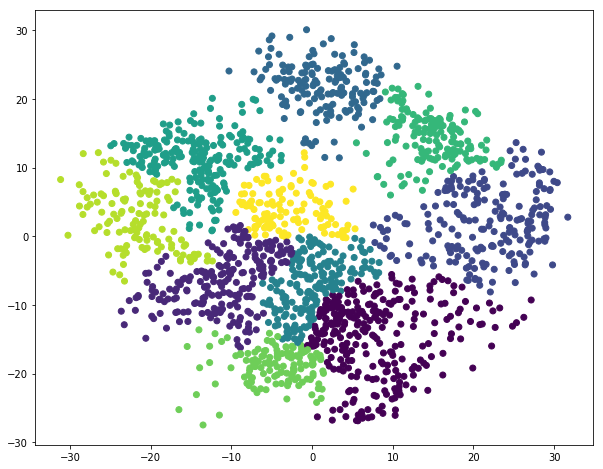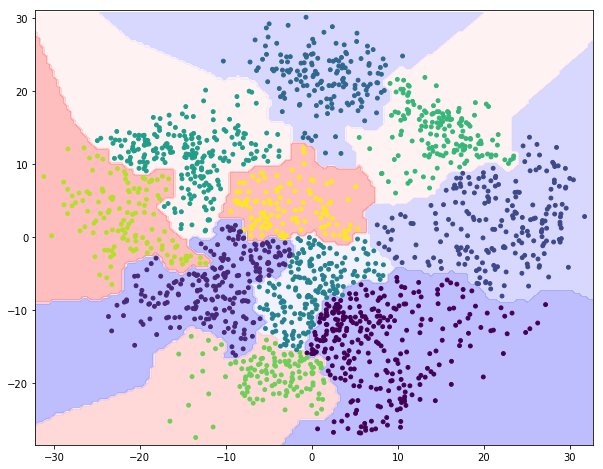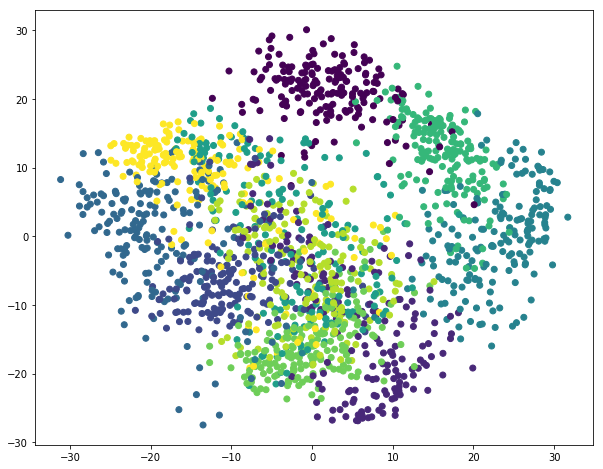array([7, 9, 4, ..., 4, 1, 4])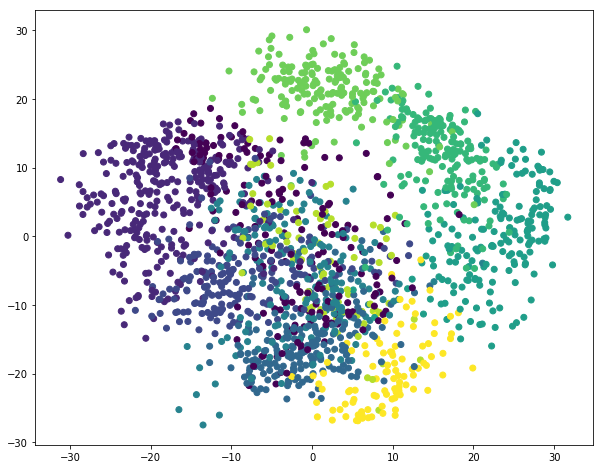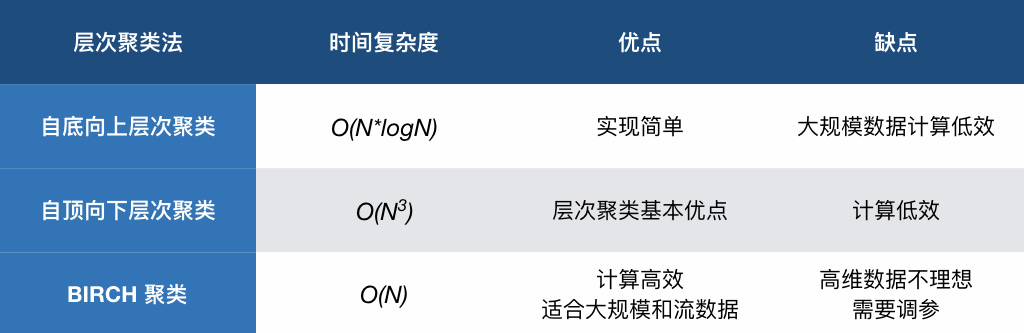### 评论

###### Your browser is out-of-date!

Update your browser to view this website correctly. Update my browser now

×